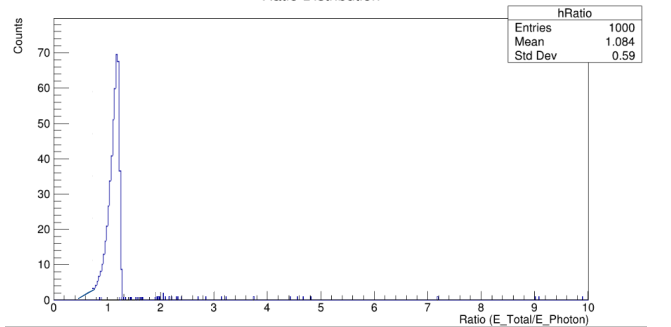# Division of two histograms

Dear Experts

I would like to divide two histograms h2 by h1. Following two histograms which I am dividing are as follows in the order of h1 and h2. My ROOT macro (where I am trying to divide them) is also attached along with the two Root files. Please check if the method I am following is correct as the output histogram should be close to 1 with mean value around 0.999

Please fill also the fields below. Note that root -b -q will tell you this info, and starting from 6.28/06 upwards, you can call .forum bug from the ROOT prompt to pre-populate a topic.

ROOT Version: Not Provided
Platform: Not Provided
Compiler: Not Provided

draw_Performance.C (2.5 KB)
bremge2v.root (516.8 KB)
totdeposit.root (3.8 KB)

The code seems ok. Maybe Divide does not do what you think/want; see the source code for Divide.

I also checked the resulting histogram everything is correct.
Since h1 and h2 are more or less the same you obtain a flat distribution with height close to 1.
And for a flat distribution like yours you have for mean and std dev.

\mu=\frac{b-a}{2}\sim \frac{10-0}{2}\sim5\\ \sigma=\sqrt{\frac{b^2-a^2}{12}}\sim\sqrt{\frac{10^2-0^2}{12}}\sim2.89

And the statistics of the histogram you obtain match with these numbers.

Maybe you are referring to the distribution of the histogram bins content that should have a mean close to 1.

Actually the output histogram should be like the following after taking the ratio between total energy and photon energyI think you are confused about how Divide works. What it does is that it takes the content of bin #1 of one histogram, divides it by the bin content of bin #1 of another histogram and puts this ratio in bin #1 of a new histogram. Rinse and repeat for all bins.

However, what you want is probably dividing the x-values of two histograms. But this is not what Divide does.

Thanks kindly suggest how I can divide x-values of two histograms

How would you do that by hand, if you didn’t have software? I don’t know what that means exactly, and maybe you don’t either. Perhaps yus can give you the code to what s/he meant by that, but you will not have learnt much (then again, maybe that’s fine with you, but I think it’s better to learn something). My suggestion: first of all, think about it, come up with a well-defined problem and a well-defined solution, how you would do it by hand (test it if you must), and then see if there’s already a way to do that in ROOT, or write the code yourself. As it is now, I don’t think you have expressed clearly what you are trying to do and how. You first said “I would like to divide two histograms h2 by h1”; and it would seem that Divide does something like that, but depends on what you really mean, so…

This topic was automatically closed 14 days after the last reply. New replies are no longer allowed.